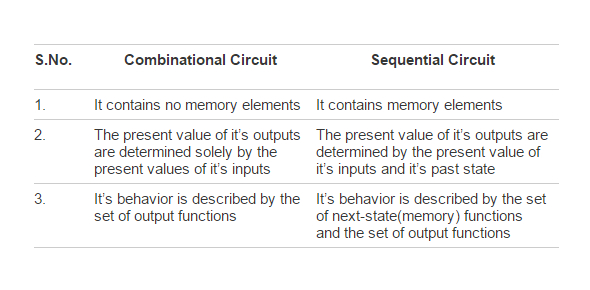# Combinational And Sequential Circuits

20 Questions | Attempts: 1522
ShareSettingsThis is a quiz for Grade 12 to help them test their knowledge on module 4

• 1.
A ____________ gate can be used to form all other gates
• 2.
When we ''Set' a latch we make Q = ________?
• A.

1

• B.

0

• C.

Invalid

• 3.
XOR and XNOR are referred to as
• A.

Sequential logic circuits

• B.

Combinational logic circuits

• C.

Basic logic gates

• D.

Complex logic gates

• 4.
For an XOR gate having A,B as inputs and Y as output mark the incorrect entry
• A.

If A =1 and B = 1 then Y = 0

• B.

If A =0 and B = 1 then Y = 1

• C.

If A =1 and B = 0 then Y = 0

• D.

If A =0 and B = 0 then Y = 1

• 5.
is the logical function for
• A.

XNOR

• B.

NOR

• C.

NAND

• D.

XOR

• 6.
A logic device that changes its output state in response to a HIGH or LOW  level  of the clock signal.
• A.

EDGE triggered latch

• B.

LEVEL triggered latch

• C.

XOR gate

• D.

NOR GATE

• 7.
1. A ___________ latch is used to eliminate the undesirable invalid state occurs in the S-R latch :
• A.

Clocked S-R latch

• B.

D latch

• C.

Edge triggered S-R latch

• D.

Multiplexer

• 8.
Latches and flip-flops  are examples of sequential circuits
• A.

True

• B.

False

• 9.
A D latch can have both Q and Q BAR  the same
• A.

True

• B.

False

• 10.
A JK-FF has no Invalid State
• A.

True

• B.

False

• 11.
To set a latch mean to make its output Q low
• A.

True

• B.

False

• 12.
What combination of R and S would lead to an invalid state?
• A.

R = 0 S = 0

• B.

R = 1 S = 0

• C.

R = 1 S = 1

• D.

R = 0 S = 1

• 13.
How does the NOT gate in a D flip flop help?
• A.

By making both S and R have the same value

• B.

By making both S and R have the opposite values

• C.

None of the above

• 14.
A latch i called BISTABLE because it has stable states.: ( More than 1 correct answer)
• A.

Q = 0

• B.

Q NOT =1

• C.

Q =1 and Q = 0

• D.

Q =HIGH and Q =LOW

• 15.
Both latches and flip-flops are circuit elements whose output depends not only on the current inputs, but also on previous inputs and outputs.
• A.

True

• B.

False

• 16.
A negative edge in a clock signal means the voltage:
• A.

Goes from 0 to 5 volts

• B.

Goes from 5 to 0 volts

• C.

Remains at 0

• D.

Remains at 5 volts

• 17.
In a clocked SR latch the outputs of the latch change when:
• A.

Clock is 0

• B.

Clock is 1

• C.

Does not depend on clock

• D.

None of the above

• 18.
This is the logic symbol of
• A.

Clocked SR latch

• B.

Edge triggered JK flip flop

• C.

JK flip flop

• D.

D flip flop

• 19.
A positive edge in a clock signal means the voltage:
• A.

Goes from 0 to 5 volts

• B.

Goes from 5 to 0 volts

• C.

Remains at 5 volts

• D.

Remains at 0 volts

• 20.
What type of logic gate can be used in a staicase switch?
• A.

AND

• B.

OR

• C.

NAND

• D.

XOR

## Related TopicsBack to top
×

Wait!
Here's an interesting quiz for you.